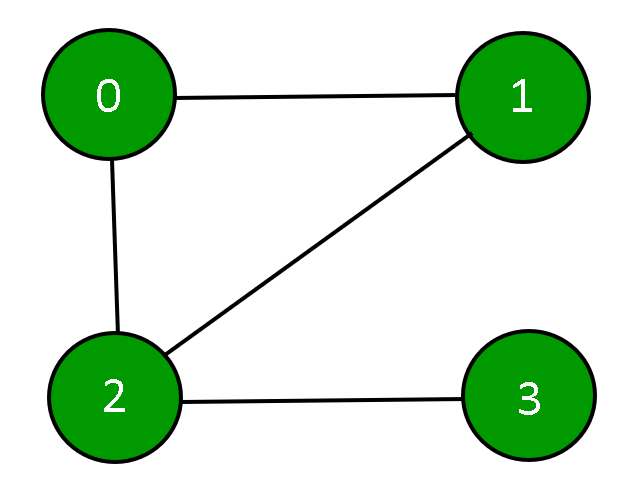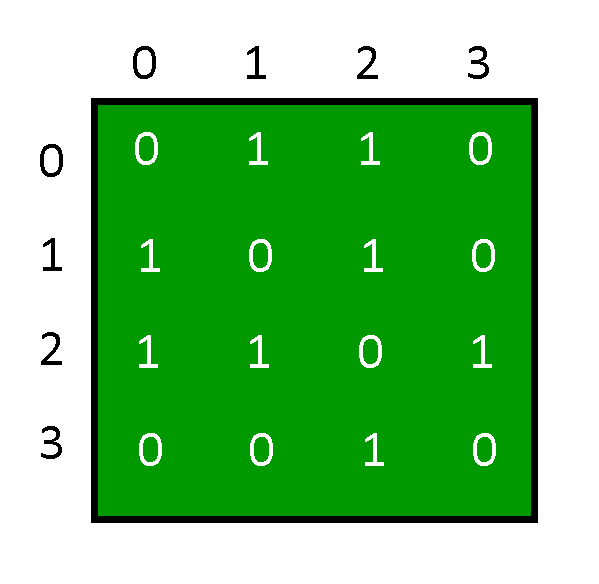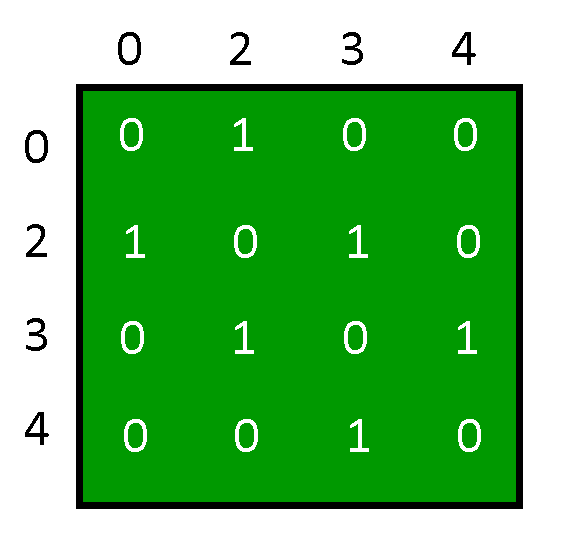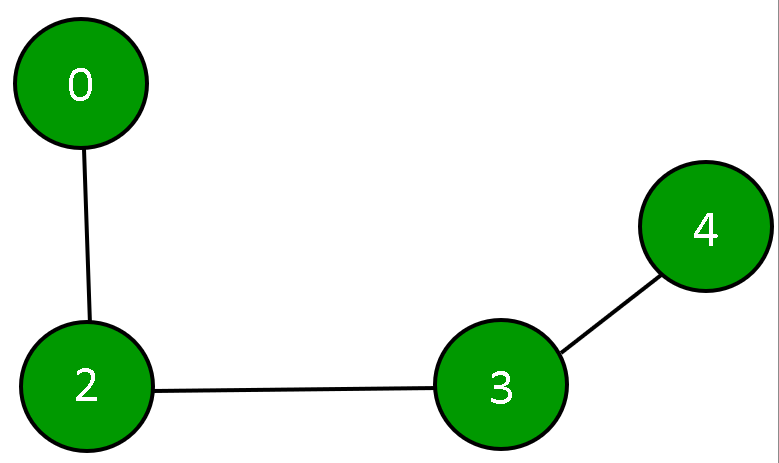A graph is a presentation of a set of entities where some pairs of entities are linked by a connection. Interconnected entities are represented by points referred to as vertices, and the connections between the vertices are termed as edges. Formally, a graph is a pair of sets (V, E), where V is a collection of vertices, and E is a collection of edges joining a pair of vertices.A graph can be represented by using an Adjacency Matrix.Initialization of Graph: The adjacency matrix will be depicted using a 2D array, a constructor will be used to assign the size of the array and each element of that array will be initialized to 0. Showing that the degree of each vertex in the graph is zero.

## C++

 `class` `Graph { ` `private``: ` `    ``// number of vertices ` `    ``int` `n; ` ` `  `    ``// adjacency matrix ` `    ``int` `g; ` ` `  `public``: ` `    ``// constructor ` `    ``Graph(``int` `x) ` `    ``{ ` `        ``n = x; ` ` `  `        ``// initializing each element of the adjacency matrix to zero ` `        ``for` `(``int` `i = 0; i < n; ++i) { ` `            ``for` `(``int` `j = 0; j < n; ++j) { ` `                ``g[i][j] = 0; ` `            ``} ` `        ``} ` `    ``} ` `}; `

## Python

 `class` `Graph: ` `     ``# number of vertices ` `     ``__n ``=` `0` ` `  `     ``# adjacency matrix ` `     ``__g ``=``[[``0` `for` `x ``in` `range``(``10``)] ``for` `y ``in` `range``(``10``)] ` `      `  `     ``# constructor ` `     ``def` `__init__(``self``, x): ` `        ``self``.__n ``=` `x ` ` `  `        ``# initializing each element of the adjacency matrix to zero ` `        ``for` `i ``in` `range``(``0``, ``self``.__n): ` `            ``for` `j ``in` `range``(``0``, ``self``.__n): ` `                ``self``.__g[i][j]``=` `0`

## Java

 `class` `Graph { ` `    ``// number of vertices ` `    ``private` `int` `n; ` ` `  `    ``// adjacency matrix ` `    ``private` `int``[][] g = ``new` `int``[``10``][``10``]; ` ` `  `    ``// constructor ` `    ``Graph(``int` `x) ` `    ``{ ` `        ``this``.n = x; ` `        ``int` `i, j; ` ` `  `        ``// initializing each element of the adjacency matrix to zero ` `        ``for` `(i = ``0``; i < n; ++i) { ` `            ``for` `(j = ``0``; j < n; ++j) { ` `                ``g[i][j] = ``0``; ` `            ``} ` `        ``} ` `    ``} ` `} `

Here the adjacency matrix is `g[n][n]` in which the degree of each vertex is zero.

Displaying the Graph: The graph is depicted using the adjacency matrix `g[n][n]` having the number of vertices n. The 2D array(adjacency matrix) is displayed in which if there is an edge between two vertices ‘x’ and ‘y’ then `g[x][y]` is 1 otherwise 0.

## C++

 `void` `displayAdjacencyMatrix() ` `{ ` `    ``cout << ``"\n\n Adjacency Matrix:"``; ` ` `  `    ``// displaying the 2D array ` `    ``for` `(``int` `i = 0; i < n; ++i) { ` `        ``cout << ``"\n"``; ` `        ``for` `(``int` `j = 0; j < n; ++j) { ` `            ``cout << ``" "` `<< g[i][j]; ` `        ``} ` `    ``} ` `} `

## Python

 `def` `displayAdjacencyMatrix(``self``): ` `        ``print``(``"\n\n Adjacency Matrix:"``, end ``=``"") ` `         `  `        ``# displaying the 2D array ` `        ``for` `i ``in` `range``(``0``, ``self``.__n): ` `            ``print``() ` `            ``for` `j ``in` `range``(``0``, ``self``.__n): ` `                ``print``("``", self.__g[i][j], end ="``") `

## Java

 `public` `void` `displayAdjacencyMatrix() ` `{ ` `    ``System.out.print(``"\n\n Adjacency Matrix:"``); ` ` `  `    ``// displaying the 2D array ` `    ``for` `(``int` `i = ``0``; i < n; ++i) { ` `        ``System.out.println(); ` `        ``for` `(``int` `j = ``0``; j < n; ++j) { ` `            ``System.out.print(``" "` `+ g[i][j]); ` `        ``} ` `    ``} ` `} `

The above method is a public member function of the class Graph which displays the graph using an adjacency matrix.

Adding Edges between Vertices in the Graph: To add edges between two existing vertices such as vertex ‘x’ and vertex ‘y’ then the elements `g[x][y]` and `g[y][x]` of the adjacency matrix will be assigned to 1, depicting that there is an edge between vertex ‘x’ and vertex ‘y’.

## C++

 `void` `addEdge(``int` `x, ``int` `y) ` `{ ` ` `  `    ``// checks if the vertex exists in the graph ` `    ``if` `((x >= n) || (y > n)) { ` `        ``cout << ``"Vertex does not exists!"``; ` `    ``} ` ` `  `    ``// checks if the vertex is connecting to itself ` `    ``if` `(x == y) { ` `        ``cout << ``"Same Vertex!"``; ` `    ``} ` `    ``else` `{ ` `        ``// connecting the vertices ` `        ``g[y][x] = 1; ` `        ``g[x][y] = 1; ` `    ``} ` `} `

## Python

 `def` `addEdge(``self``, x, y): `   `        ``# checks if the vertex exists in the graph  ` `        ``if``(x>``=` `self``.__n) ``or` `(y >``=` `self``.__n): ` `            ``print``(``"Vertex does not exists !"``) ` `         `  `        ``# checks if the vertex is connecting to itself ` `        ``if``(x ``=``=` `y): ` `             ``print``(``"Same Vertex !"``) ` `        ``else``: ` `              `  `             ``# connecting the vertices ` `             ``self``.__g[y][x]``=` `1` `             ``self``.__g[x][y]``=` `1`

## Java

 `public` `void` `addEdge(``int` `x, ``int` `y) ` `{ ` `    ``// checks if the vertex exists in the graph ` `    ``if` `((x >= n) || (y > n)) { ` `        ``System.out.println(``"Vertex does not exists!"``); ` `    ``} ` ` `  `    ``// checks if the vertex is connecting to itself ` `    ``if` `(x == y) { ` `        ``System.out.println(``"Same Vertex!"``); ` `    ``} ` `    ``else` `{ ` `        ``// connecting the vertices ` `        ``g[y][x] = ``1``; ` `        ``g[x][y] = ``1``; ` `    ``} ` `} `

Here the above method is a public member function of the class Graph which connects any two existing vertices in the Graph.

Adding a Vertex in the Graph: To add a vertex in the graph, we need to increase both the row and column of the existing adjacency matrix and then initialize the new elements related to that vertex to 0.(i.e the new vertex added is not connected to any other vertex)

## C++

 `void` `addVertex() ` `{ ` `    ``// increasing the number of vertices ` `    ``n++; ` `    ``int` `i; ` ` `  `    ``// initializing the new elements to 0 ` `    ``for` `(i = 0; i < n; ++i) { ` `        ``g[i][n - 1] = 0; ` `        ``g[n - 1][i] = 0; ` `    ``} ` `} `

## Python

 `def` `addVertex(``self``): ` `          `  `         ``# increasing the number of vertices ` `         ``self``.__n ``=` `self``.__n ``+` `1``; ` `          `  `         ``# initializing the new elements to 0  ` `         ``for` `i ``in` `range``(``0``, ``self``.__n): ` `             ``self``.__g[i][``self``.__n``-``1``]``=` `0` `             ``self``.__g[``self``.__n``-``1``][i]``=` `0`

## java

 `public` `void` `addVertex() ` `{ ` `    ``// increasing the number of vertices ` `    ``n++; ` `    ``int` `i; ` ` `  `    ``// initializing the new elements to 0 ` `    ``for` `(i = ``0``; i < n; ++i) { ` `        ``g[i][n - ``1``] = ``0``; ` `        ``g[n - ``1``][i] = ``0``; ` `    ``} ` `} `

The above method is a public member function of the class Graph which increments the number of vertices by 1 and the degree of the new vertex is 0.

Removing a Vertex in the Graph: To remove a vertex from the graph, we need to check if that vertex exists in the graph or not and if that vertex exists then we need to shift the rows to the left and the columns upwards of the adjacency matrix so that the row and column values of the given vertex gets replaced by the values of the next vertex and then decrease the number of vertices by 1.In this way that particular vertex will be removed from the adjacency matrix.

## C++

 `void` `removeVertex(``int` `x) ` `{ ` `    ``// checking if the vertex is present ` `    ``if` `(x > n) { ` `        ``cout << ``"\nVertex not present!"``; ` `        ``return``; ` `    ``} ` `    ``else` `{ ` `        ``int` `i; ` ` `  `        ``// removing the vertex ` `        ``while` `(x < n) { ` `            ``// shifting the rows to left side ` `            ``for` `(i = 0; i < n; ++i) { ` `                ``g[i][x] = g[i][x + 1]; ` `            ``} ` ` `  `            ``// shifting the columns upwards ` `            ``for` `(i = 0; i < n; ++i) { ` `                ``g[x][i] = g[x + 1][i]; ` `            ``} ` `            ``x++; ` `        ``} ` ` `  `        ``// decreasing the number of vertices ` `        ``n--; ` `    ``} ` `} `

## Python

 `def` `removeVertex(``self``, x): ` `         `  `        ``# checking if the vertex is present ` `        ``if``(x>``self``.__n): ` `            ``print``(``"Vertex not present !"``) ` `        ``else``: ` `         `  `          ``# removing the vertex ` `          ``while``(x<``self``.__n): ` `         `  `             ``# shifting the rows to left side  ` `             ``for` `i ``in` `range``(``0``, ``self``.__n): ` `                  ``self``.__g[i][x]``=` `self``.__g[i][x ``+` `1``] ` `            `  `             ``# shifting the columns upwards ` `             ``for` `i ``in` `range``(``0``, ``self``.__n): ` `                  ``self``.__g[x][i]``=` `self``.__g[x ``+` `1``][i] ` `             ``x ``=` `x ``+` `1` ` `  `          ``# decreasing the number of vertices ` `          ``self``.__n ``=` `self``.__n ``-` `1`

## Java

 `public` `void` `removeVertex(``int` `x) ` `{ ` `    ``// checking if the vertex is present ` `    ``if` `(x > n) { ` `        ``System.out.println(``"Vertex not present!"``); ` `        ``return``; ` `    ``} ` `    ``else` `{ ` `        ``int` `i; ` ` `  `        ``// removing the vertex ` `        ``while` `(x < n) { ` ` `  `            ``// shifting the rows to left side ` `            ``for` `(i = ``0``; i < n; ++i) { ` `                ``g[i][x] = g[i][x + ``1``]; ` `            ``} ` ` `  `            ``// shifting the columns upwards ` `            ``for` `(i = ``0``; i < n; ++i) { ` `                ``g[x][i] = g[x + ``1``][i]; ` `            ``} ` `            ``x++; ` `        ``} ` ` `  `        ``// decreasing the number of vertices ` `        ``n--; ` `    ``} ` `} `

The above method is a public member function of the class Graph which removes an existing vertex from the graph by shifting the rows to the left and shifting the columns up to replace the row and column values of that vertex with the next vertex and then decreases the number of vertices by 1 in the graph.

Following is a complete program that uses all of the above methods in a Graph.

## C++

 `// C++ program to add and remove Vertex in Adjacency Matrix ` ` `  `#include ` ` `  `using` `namespace` `std; ` ` `  `class` `Graph { ` `private``: ` `    ``// number of vertices ` `    ``int` `n; ` ` `  `    ``// adjacency matrix ` `    ``int` `g; ` ` `  `public``: ` `    ``// constructor ` `    ``Graph(``int` `x) ` `    ``{ ` `        ``n = x; ` ` `  `        ``// initializing each element of the adjacency matrix to zero ` `        ``for` `(``int` `i = 0; i < n; ++i) { ` `            ``for` `(``int` `j = 0; j < n; ++j) { ` `                ``g[i][j] = 0; ` `            ``} ` `        ``} ` `    ``} ` ` `  `    ``void` `displayAdjacencyMatrix() ` `    ``{ ` `        ``cout << ``"\n\n Adjacency Matrix:"``; ` ` `  `        ``// displaying the 2D array ` `        ``for` `(``int` `i = 0; i < n; ++i) { ` `            ``cout << ``"\n"``; ` `            ``for` `(``int` `j = 0; j < n; ++j) { ` `                ``cout << ``" "` `<< g[i][j]; ` `            ``} ` `        ``} ` `    ``} ` ` `  `    ``void` `addEdge(``int` `x, ``int` `y) ` `    ``{ ` ` `  `        ``// checks if the vertex exists in the graph ` `        ``if` `((x >= n) || (y > n)) { ` `            ``cout << ``"Vertex does not exists!"``; ` `        ``} ` ` `  `        ``// checks if the vertex is connecting to itself ` `        ``if` `(x == y) { ` `            ``cout << ``"Same Vertex!"``; ` `        ``} ` `        ``else` `{ ` `            ``// connecting the vertices ` `            ``g[y][x] = 1; ` `            ``g[x][y] = 1; ` `        ``} ` `    ``} ` ` `  `    ``void` `addVertex() ` `    ``{ ` `        ``// increasing the number of vertices ` `        ``n++; ` `        ``int` `i; ` ` `  `        ``// initializing the new elements to 0 ` `        ``for` `(i = 0; i < n; ++i) { ` `            ``g[i][n - 1] = 0; ` `            ``g[n - 1][i] = 0; ` `        ``} ` `    ``} ` ` `  `    ``void` `removeVertex(``int` `x) ` `    ``{ ` `        ``// checking if the vertex is present ` `        ``if` `(x > n) { ` `            ``cout << ``"\nVertex not present!"``; ` `            ``return``; ` `        ``} ` `        ``else` `{ ` `            ``int` `i; ` ` `  `            ``// removing the vertex ` `            ``while` `(x < n) { ` `                ``// shifting the rows to left side ` `                ``for` `(i = 0; i < n; ++i) { ` `                    ``g[i][x] = g[i][x + 1]; ` `                ``} ` ` `  `                ``// shifting the columns upwards ` `                ``for` `(i = 0; i < n; ++i) { ` `                    ``g[x][i] = g[x + 1][i]; ` `                ``} ` `                ``x++; ` `            ``} ` ` `  `            ``// decreasing the number of vertices ` `            ``n--; ` `        ``} ` `    ``} ` `}; ` ` `  `int` `main() ` `{ ` `    ``// creating objects of class Graph ` `    ``Graph obj(4); ` ` `  `    ``// calling methods ` `    ``obj.addEdge(0, 1); ` `    ``obj.addEdge(0, 2); ` `    ``obj.addEdge(1, 2); ` `    ``obj.addEdge(2, 3); ` `    ``// the adjacency matrix created ` `    ``obj.displayAdjacencyMatrix(); ` ` `  `    ``// adding a vertex to the graph ` `    ``obj.addVertex(); ` `    ``// connecting that verex to other existing vertices ` `    ``obj.addEdge(4, 1); ` `    ``obj.addEdge(4, 3); ` `    ``// the adjacency matrix with a new vertex ` `    ``obj.displayAdjacencyMatrix(); ` ` `  `    ``// removing an existing vertex in the graph ` `    ``obj.removeVertex(1); ` `    ``// the adjacency matrix after removing a vertex ` `    ``obj.displayAdjacencyMatrix(); ` ` `  `    ``return` `0; ` `} `

## Java

 `// Java program to add and remove Vertex in Adjacency Matrix ` `class` `Graph ` `{ ` `    ``// number of vertices ` `    ``private` `int` `n; ` ` `  `    ``// adjacency matrix ` `    ``private` `int``[][] g = ``new` `int``[``10``][``10``]; ` ` `  `    ``// constructor ` `    ``Graph(``int` `x) ` `    ``{ ` `        ``this``.n = x; ` `        ``int` `i, j; ` ` `  `        ``// initializing each element of  ` `        ``// the adjacency matrix to zero ` `        ``for` `(i = ``0``; i < n; ++i)  ` `        ``{ ` `            ``for` `(j = ``0``; j < n; ++j)  ` `            ``{ ` `                ``g[i][j] = ``0``; ` `            ``} ` `        ``} ` `    ``} ` ` `  `    ``public` `void` `displayAdjacencyMatrix() ` `    ``{ ` `        ``System.out.print(``"\n\n Adjacency Matrix:"``); ` ` `  `        ``// displaying the 2D array ` `        ``for` `(``int` `i = ``0``; i < n; ++i) ` `        ``{ ` `            ``System.out.println(); ` `            ``for` `(``int` `j = ``0``; j < n; ++j)  ` `            ``{ ` `                ``System.out.print(``" "` `+ g[i][j]); ` `            ``} ` `        ``} ` `    ``} ` ` `  `    ``public` `void` `addEdge(``int` `x, ``int` `y) ` `    ``{ ` `        ``// checks if the vertex exists in the graph ` `        ``if` `((x >= n) || (y > n)) ` `        ``{ ` `            ``System.out.println(``"Vertex does not exists!"``); ` `        ``} ` ` `  `        ``// checks if the vertex is connecting to itself ` `        ``if` `(x == y)  ` `        ``{ ` `            ``System.out.println(``"Same Vertex!"``); ` `        ``} ` `        ``else`  `        ``{ ` `            ``// connecting the vertices ` `            ``g[y][x] = ``1``; ` `            ``g[x][y] = ``1``; ` `        ``} ` `    ``} ` ` `  `    ``public` `void` `addVertex() ` `    ``{ ` `        ``// increasing the number of vertices ` `        ``n++; ` `        ``int` `i; ` ` `  `        ``// initializing the new elements to 0 ` `        ``for` `(i = ``0``; i < n; ++i) ` `        ``{ ` `            ``g[i][n - ``1``] = ``0``; ` `            ``g[n - ``1``][i] = ``0``; ` `        ``} ` `    ``} ` ` `  `    ``public` `void` `removeVertex(``int` `x) ` `    ``{ ` `        ``// checking if the vertex is present ` `        ``if` `(x > n)  ` `        ``{ ` `            ``System.out.println(``"Vertex not present!"``); ` `            ``return``; ` `        ``} ` `        ``else`  `        ``{ ` `            ``int` `i; ` ` `  `            ``// removing the vertex ` `            ``while` `(x < n) ` `            ``{ ` ` `  `                ``// shifting the rows to left side ` `                ``for` `(i = ``0``; i < n; ++i)  ` `                ``{ ` `                    ``g[i][x] = g[i][x + ``1``]; ` `                ``} ` ` `  `                ``// shifting the columns upwards ` `                ``for` `(i = ``0``; i < n; ++i) ` `                ``{ ` `                    ``g[x][i] = g[x + ``1``][i]; ` `                ``} ` `                ``x++; ` `            ``} ` ` `  `            ``// decreasing the number of vertices ` `            ``n--; ` `        ``} ` `    ``} ` `} ` ` `  `class` `Main  ` `{ ` `    ``public` `static` `void` `main(String[] args) ` `    ``{ ` `        ``// creating objects of class Graph ` `        ``Graph obj = ``new` `Graph(``4``); ` ` `  `        ``// calling methods ` `        ``obj.addEdge(``0``, ``1``); ` `        ``obj.addEdge(``0``, ``2``); ` `        ``obj.addEdge(``1``, ``2``); ` `        ``obj.addEdge(``2``, ``3``); ` `         `  `        ``// the adjacency matrix created ` `        ``obj.displayAdjacencyMatrix(); ` ` `  `        ``// adding a vertex to the graph ` `        ``obj.addVertex(); ` `         `  `        ``// connecting that verex to other existing vertices ` `        ``obj.addEdge(``4``, ``1``); ` `        ``obj.addEdge(``4``, ``3``); ` `         `  `        ``// the adjacency matrix with a new vertex ` `        ``obj.displayAdjacencyMatrix(); ` ` `  `        ``// removing an existing vertex in the graph ` `        ``obj.removeVertex(``1``); ` `         `  `        ``// the adjacency matrix after removing a vertex ` `        ``obj.displayAdjacencyMatrix(); ` `    ``} ` `} `

## Python

 `# Python program to add and remove Vertex in Adjacency Matrix ` ` `  `class` `Graph: ` `     ``# number of vertices ` `     ``__n ``=` `0` `  `  `     ``# adjacency matrix ` `     ``__g ``=``[[``0` `for` `x ``in` `range``(``10``)] ``for` `y ``in` `range``(``10``)] ` `       `  `     ``# constructor ` `     ``def` `__init__(``self``, x): ` `        ``self``.__n ``=` `x ` `  `  `        ``# initializing each element of the adjacency matrix to zero ` `        ``for` `i ``in` `range``(``0``, ``self``.__n): ` `            ``for` `j ``in` `range``(``0``, ``self``.__n): ` `                ``self``.__g[i][j]``=` `0` ` `  `     ``def` `displayAdjacencyMatrix(``self``): ` `        ``print``(``"\n\n Adjacency Matrix:"``, end ``=``"") ` `         `  `        ``# displaying the 2D array ` `        ``for` `i ``in` `range``(``0``, ``self``.__n): ` `            ``print``() ` `            ``for` `j ``in` `range``(``0``, ``self``.__n): ` `                ``print``("``", self.__g[i][j], end ="``") ` `     ``def` `addEdge(``self``, x, y): ` ` `  `        ``# checks if the vertex exists in the graph  ` `        ``if``(x>``=` `self``.__n) ``or` `(y >``=` `self``.__n): ` `            ``print``(``"Vertex does not exists !"``) ` `          `  `        ``# checks if the vertex is connecting to itself ` `        ``if``(x ``=``=` `y): ` `             ``print``(``"Same Vertex !"``) ` `        ``else``: ` `               `  `             ``# connecting the vertices ` `             ``self``.__g[y][x]``=` `1` `             ``self``.__g[x][y]``=` `1`       ` `  `     ``def` `addVertex(``self``): ` `           `  `         ``# increasing the number of vertices ` `         ``self``.__n ``=` `self``.__n ``+` `1``; ` `           `  `         ``# initializing the new elements to 0  ` `         ``for` `i ``in` `range``(``0``, ``self``.__n): ` `             ``self``.__g[i][``self``.__n``-``1``]``=` `0` `             ``self``.__g[``self``.__n``-``1``][i]``=` `0`                   `     ``def` `removeVertex(``self``, x): ` `          `  `        ``# checking if the vertex is present ` `        ``if``(x>``self``.__n): ` `             ``print``(``"Vertex not present !"``) ` `        ``else``: ` `          `  `             ``# removing the vertex ` `             ``while``(x<``self``.__n): ` `          `  `                 ``# shifting the rows to left side  ` `                 ``for` `i ``in` `range``(``0``, ``self``.__n): ` `                       ``self``.__g[i][x]``=` `self``.__g[i][x ``+` `1``] ` `             `  `                 ``# shifting the columns upwards ` `                 ``for` `i ``in` `range``(``0``, ``self``.__n): ` `                       ``self``.__g[x][i]``=` `self``.__g[x ``+` `1``][i] ` `                 ``x ``=` `x ``+` `1` `  `  `             ``# decreasing the number of vertices ` `             ``self``.__n ``=` `self``.__n ``-` `1`              ` `  ` `  `# creating objects of class Graph  ` `obj ``=` `Graph(``4``); ` `      `  `# calling methods ` `obj.addEdge(``0``, ``1``); ` `obj.addEdge(``0``, ``2``); ` `obj.addEdge(``1``, ``2``); ` `obj.addEdge(``2``, ``3``); ` `# the adjacency matrix created ` `obj.displayAdjacencyMatrix(); ` `  `  `# adding a vertex to the graph ` `obj.addVertex(); ` `# connecting that verex to other existing vertices ` `obj.addEdge(``4``, ``1``); ` `obj.addEdge(``4``, ``3``); ` `# the adjacency matrix with a new vertex ` `obj.displayAdjacencyMatrix(); ` `      `  `# removing an existing vertex in the graph ` `obj.removeVertex(``1``); ` `# the adjacency matrix after removing a vertex ` `obj.displayAdjacencyMatrix(); `

## C#

 `// C# program to add and remove Vertex in Adjacency Matrix ` `using` `System; ` ` `  `public` `class` `Graph  ` `{ ` `    ``// number of vertices ` `    ``private` `int` `n; ` ` `  `    ``// adjacency matrix ` `    ``private` `int``[,] g = ``new` `int``[10, 10]; ` ` `  `    ``// constructor ` `    ``public` `Graph(``int` `x) ` `    ``{ ` `        ``this``.n = x; ` `        ``int` `i, j; ` ` `  `        ``// initializing each element of the adjacency matrix to zero ` `        ``for` `(i = 0; i < n; ++i) ` `        ``{ ` `            ``for` `(j = 0; j < n; ++j)  ` `            ``{ ` `                ``g[i, j] = 0; ` `            ``} ` `        ``} ` `    ``} ` ` `  `    ``public` `void` `displayAdjacencyMatrix() ` `    ``{ ` `        ``Console.Write(``"\n\n Adjacency Matrix:"``); ` ` `  `        ``// displaying the 2D array ` `        ``for` `(``int` `i = 0; i < n; ++i) ` `        ``{ ` `            ``Console.WriteLine(); ` `            ``for` `(``int` `j = 0; j < n; ++j)  ` `            ``{ ` `                ``Console.Write(``" "` `+ g[i, j]); ` `            ``} ` `        ``} ` `    ``} ` ` `  `    ``public` `void` `addEdge(``int` `x, ``int` `y) ` `    ``{ ` `        ``// checks if the vertex exists in the graph ` `        ``if` `((x >= n) || (y > n)) ` `        ``{ ` `            ``Console.WriteLine(``"Vertex does not exists!"``); ` `        ``} ` ` `  `        ``// checks if the vertex is connecting to itself ` `        ``if` `(x == y) ` `        ``{ ` `            ``Console.WriteLine(``"Same Vertex!"``); ` `        ``} ` `        ``else` `        ``{ ` `            ``// connecting the vertices ` `            ``g[y, x] = 1; ` `            ``g[x, y] = 1; ` `        ``} ` `    ``} ` ` `  `    ``public` `void` `addVertex() ` `    ``{ ` `        ``// increasing the number of vertices ` `        ``n++; ` `        ``int` `i; ` ` `  `        ``// initializing the new elements to 0 ` `        ``for` `(i = 0; i < n; ++i) ` `        ``{ ` `            ``g[i, n - 1] = 0; ` `            ``g[n - 1, i] = 0; ` `        ``} ` `    ``} ` ` `  `    ``public` `void` `removeVertex(``int` `x) ` `    ``{ ` `        ``// checking if the vertex is present ` `        ``if` `(x > n)  ` `        ``{ ` `            ``Console.WriteLine(``"Vertex not present!"``); ` `            ``return``; ` `        ``} ` `        ``else` `        ``{ ` `            ``int` `i; ` ` `  `            ``// removing the vertex ` `            ``while` `(x < n)  ` `            ``{ ` ` `  `                ``// shifting the rows to left side ` `                ``for` `(i = 0; i < n; ++i) ` `                ``{ ` `                    ``g[i, x] = g[i, x + 1]; ` `                ``} ` ` `  `                ``// shifting the columns upwards ` `                ``for` `(i = 0; i < n; ++i)  ` `                ``{ ` `                    ``g[x, i] = g[x + 1, i]; ` `                ``} ` `                ``x++; ` `            ``} ` ` `  `            ``// decreasing the number of vertices ` `            ``n--; ` `        ``} ` `    ``} ` `} ` ` `  `public` `class` `GFG  ` `{ ` `    ``// Driver code ` `    ``public` `static` `void` `Main(String[] args) ` `    ``{ ` `        ``// creating objects of class Graph ` `        ``Graph obj = ``new` `Graph(4); ` ` `  `        ``// calling methods ` `        ``obj.addEdge(0, 1); ` `        ``obj.addEdge(0, 2); ` `        ``obj.addEdge(1, 2); ` `        ``obj.addEdge(2, 3); ` `        ``// the adjacency matrix created ` `        ``obj.displayAdjacencyMatrix(); ` ` `  `        ``// adding a vertex to the graph ` `        ``obj.addVertex(); ` `         `  `        ``// connecting that verex to other existing vertices ` `        ``obj.addEdge(4, 1); ` `        ``obj.addEdge(4, 3); ` `         `  `        ``// the adjacency matrix with a new vertex ` `        ``obj.displayAdjacencyMatrix(); ` ` `  `        ``// removing an existing vertex in the graph ` `        ``obj.removeVertex(1); ` `         `  `        ``// the adjacency matrix after removing a vertex ` `        ``obj.displayAdjacencyMatrix(); ` `    ``} ` `} ` ` `  `// This code is contributed by PrinciRaj1992 `

Output:

```Adjacency Matrix:
0 1 1 0
1 0 1 0
1 1 0 1
0 0 1 0

0 1 1 0 0
1 0 1 0 1
1 1 0 1 0
0 0 1 0 1
0 1 0 1 0

0 1 0 0
1 0 1 0
0 1 0 1
0 0 1 0```

Adjacency matrices wastes lot of memory space. Such matrices are found to be very sparse.This representation requires space for n*n elements, the time complexity of `addVertex()` method is O(n) and the time complexity of `removeVertex()` method is O(n*n) for a graph of n vertices.
From the output of the program, the Adjacency Matrix is:And the Graph depicted by the above Adjacecny Matrix is:GeeksforGeeks has prepared a complete interview preparation course with premium videos, theory, practice problems, TA support and many more features. Please refer Placement 100 for details

My Personal Notes arrow_drop_upCheck out this Author's contributed articles.

If you like GeeksforGeeks and would like to contribute, you can also write an article using contribute.geeksforgeeks.org or mail your article to contribute@geeksforgeeks.org. See your article appearing on the GeeksforGeeks main page and help other Geeks.

Please Improve this article if you find anything incorrect by clicking on the "Improve Article" button below.

Improved By : princiraj1992

Article Tags :
Practice Tags :

1

Please write to us at contribute@geeksforgeeks.org to report any issue with the above content.